Next: Jerrold Griggs - Extremal Up: Extremal Combinatorics / Combinatoire Previous: Jason Brown - The

## David Fisher - The minimum number of triangles in a graph

 DAVID FISHER, Department of Mathematics, University of Colorado at Denver, Denver, Colorado  80217-3364, USA The minimum number of triangles in a graph

Given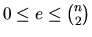, what is the minimum number of triangles in a graph with n vertices and e edges? Let tn,ebe the answer. For example, G has 7 vertices, 13 edges, and 3triangles. =.12in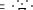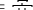Since any graph with 7 vertices and 13 edges has at least 3triangles, we have t7,13=3.

Trivially, tn,e=0 if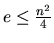. McKay (1963) proved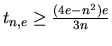. Bollobás (1978) improved this by showing tn,e is at least the piecewise linear function with nodes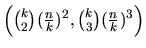for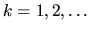. Fisher (1989) found a better bound when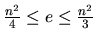by proving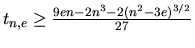. Nikiforov and Khadzhiivanov (1981) and Lovász and Simonovits (1983) independently found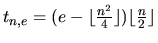for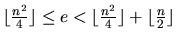.

I have a conjecture: If G has n vertices,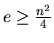edges, and tn,e triangles, then Gc is not connected. Barnhart and I verified this when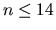. If true for all n, one can recursively find tn,e. This would give analytic proofs of all results above plus a new bound similar to Fisher's when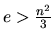.Next: Jerrold Griggs - Extremal Up: Extremal Combinatorics / Combinatoire Previous: Jason Brown - The